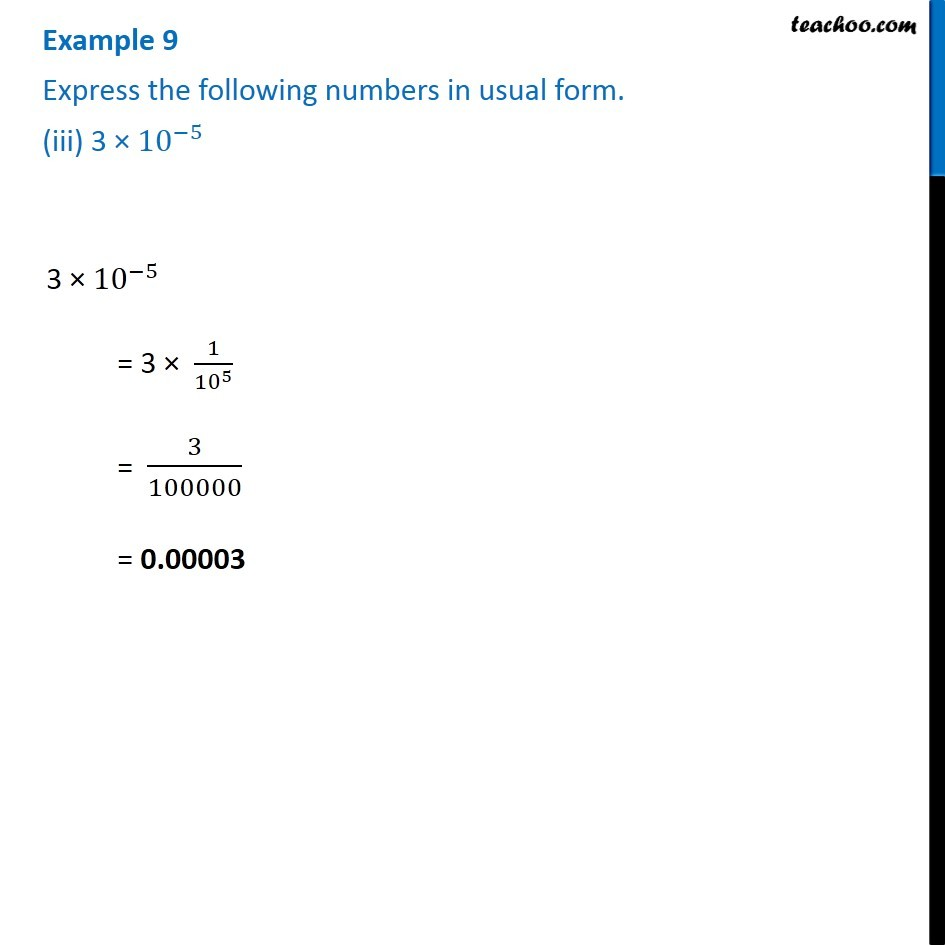1. Chapter 12 Class 8 Exponents and Powers
2. Serial order wise
3. Examples

Transcript

Example 9 Express the following numbers in usual form. (iii) 3 × 〖10〗^(−5) 3 × 〖10〗^(−5) = 3 × 1/〖10〗^5 = 3/100000 = 0.00003